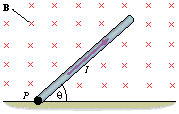# Force on a current-carrying rod

• Dart82
In summary, the angle at which the uniform magnetic field points into the plane of the paper is 33.0 degrees.f

## Homework Statement

a thin, uniform rod, which has a length of 0.45 m and a mass of 0.080 kg. This rod lies in the plane of the paper and is attached to the floor by a hinge at point P. A uniform magnetic field of 0.25 T is directed perpendicularly into the plane of the paper. There is a current I = 3.8 A in the rod, which does not rotate clockwise or counter-clockwise. Find the angle .## Homework Equations

F = ILB sin(theta)
F= ma

## The Attempt at a Solution

heres what i was thinking:
if F = ILB sin(theta) then let ma = ILBsin(theta)

so solve for theta:
arcsine(ILB/ma) = 33.0 degrees however, i was told this is wrong.Did you draw out a free body diagram? Which direction is the force acting in? Which direction is gravity acting in?

You have the right idea, but I think that you must have either skipped over drawing a free body diagram, or you made a mistake forming your similar triangles.

hmm, i guess i am confused about the orientation of the picture. i took it as i am looking at the picture as though i was looking at the top of a door (the hinge attaching it to the wall being point P ) with the magnetic field pointing into the floor. the force would then point toward the upper left corner of the picture. am i off target with this line of thinking?
Next, i would draw a right triangle on the right hand side tip of the rod downward.

[URL=[URL][PLAIN]http://imageshack.us][PLAIN]http://img76.imageshack.us/img76/8448/2135tb9.gif [Broken]

Last edited by a moderator:
Yep, this is what you do. Now you know that your EM force components and gravitational force components will cancel. It's all pretty similar to a mass on an inclined plane.

You also posted this in the advanced physics section. Why did you ignore the advice there??

You also posted this in the advanced physics section. Why did you ignore the advice there??
wasn't intending to ignore the advice, several hours had gone by without any response in the intro forum so i posted in the advanced forum to see if anyone could provide some insight. it was my last homework ?, and it needed to be turned in by the end of the hour. actually what you told me in the advanced forum, finally made things click. thanks.

Ok, but you shouldn't multiple post. If you think it's been overlooked, post another message on the same thread and that will bump it up in the queue.

not a problem

If mg=ILBsin(theta), then wouldn't theta=arcsin(mg/ILB)?

Edit: Also, L=twice what they give you for L.

Last edited:
What?? Why would you multiply the length by two?

Sure, if mg=ILBsin(theta) then algebra will show that theta=arcsin(mg/ILB). It depends on what angle you are talking about. I get that the B-force is orthogonal to the wire, and force from gravity points directly down.

Assuming the rod is uniform, all gravity components and force components will act equally on each other, so just look at one small contribution dF_b and dF_g. Make a similar triangle with the small contributions, and you find that the gravity vector normal to the plane, that cancels the B-force, has a component of mgcos(theta).

Newton's Second Law:
dF_b - d(mgcos(theta)) = 0
F_b=mgcos(theta)
cos(theta)=(F_b/mg)
theta=arccos(ILB/mg)